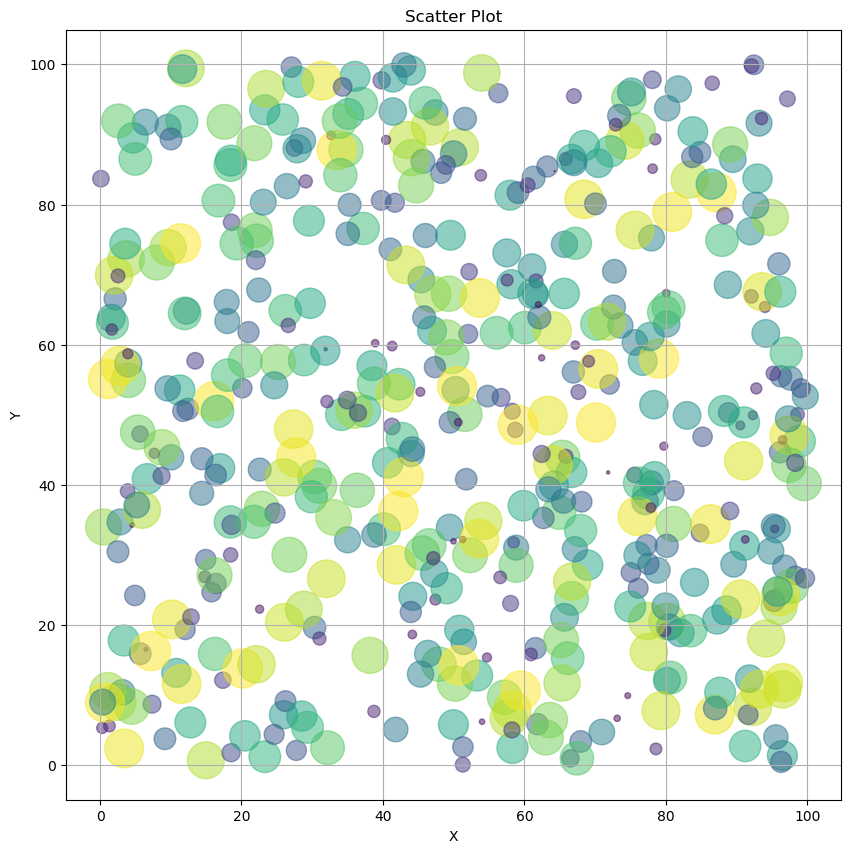This is a simple Julia 1.0 notebook.

Below we'll show off the Julia PyPlot package (JuliaPy/PyPlot.jl), which provides a Julia-language interface to python's matplotlib module.

In :
using PyPlot

In :
x = 100 * rand(500)
y = 100 * rand(500)
areas = 800 * rand(500);

In :
#  Scatterplot
fig = figure("scatterplot",figsize=(10,10))
scatter(x,y,s=areas,alpha=0.5, c=areas)

title("Scatter Plot")
xlabel("X")
ylabel("Y")
grid("on")
gcf();